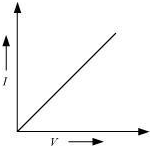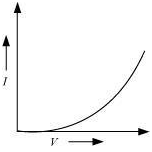1800-212-7858 (Toll Free)
9:00am - 8:00pm IST all days
8104911739

or

Thanks, You will receive a call shortly.
Customer Support

You are very important to us

022-62211530

Mon to Sat - 11 AM to 8 PM

# Wht is resitivity? and Potenial Differecces?

Asked by Vineet Chauhan 6th October 2013, 6:59 PM

1.

Resistivity is the resistance provided by a material of 1 unit length and 1 unit area.

It is denoted by the 'rho '

Factors on which resistvity depends on:

1. It is inversevly proportational to temprature

2. It depends on the nature of the material

Unit of Resistvity is Ohm Metre

Deriviation:

Resistivity = Resistance (length/area)

Resistivity = Ohm (metre/metre2) where metre and metre cancel

Resistivity = Ohm metre

2.

Potential difference is the difference in electric potential between any two points in an electric field or in a circuit.

3.

Let's begin with the Ohm's Law

It states that the amount of current (I) flowing through a material (say a wire) is directly proportional to the voltage (V) applied across its ends. Mathematically, it is given as

V ? I

or

V = RI

here R is a constant of proportionality also known as the resistance.

So, the resistance of a material following the Ohm's Law will always stay constant for all values of external voltage applied.

Now, using this principle, we can distinguish materials as either being Ohmic or Non-Ohmic in nature. Such that,

Ohmic materials follow the Ohms' law as the resistance remains unaffected even if we change the applied voltage (which alters amount of current flowing).

The V-I graph in this case shows a linear trend, as followsSo, If it is a straight line with a positive gradient then the resistor is following Ohm's law V=IR. The gradient is the component's resistance.

Non-Ohmic materials, do not obey the Ohm's Law, as the resistance varies with applied voltage.

The V-I graph in this case would show a gradual curved slope, as follows4.

Resistivity is defined as the resistance offered by a cube of a material of side 1 m when current flows perpendicular to its opposite faces. Its S.I. unit is ohm-meter (?m). Resistivity, ? = RA/L

Answered by Expert 7th October 2013, 9:25 AM
• 1
• 2
• 3
• 4
• 5
• 6
• 7
• 8
• 9
• 10

You have rated this answer /10